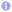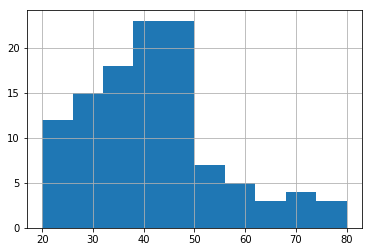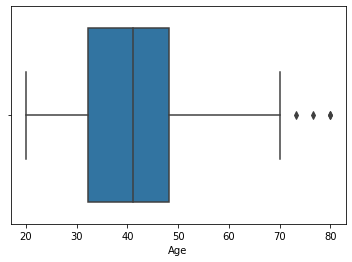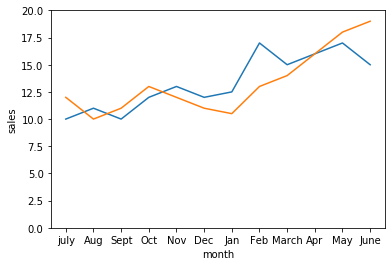Introduction to Data Visualization

Bar Chart: A bar chart or bar graph is a chart or graph that presents categorical data with rectangular bars with heights or lengths proportional to the values that they represent. The bars can be plotted vertically or horizontally. A vertical bar chart is sometimes called a line graph.

A bar graph is a nice way to display categorical data.

Below is the pictorial representation for the bar chart

 Source code```import numpy as np
import pandas as pd
import matplotlib.pyplot as plt
import seaborn as sns```
 Source code```prob={"X_axis finite discrete random variables":['x0=1','x1=5','x2=10','x3=10','x4=5','x5=1'],'col2':[1/32,5/32,10/32,10/32,5/32,1/32]}
prob=pd.DataFrame(prob)
a=sns.barplot(x='X_axis finite discrete random variables',y='col2',data=prob).set_ylabel('1/32                      5/32                        10/32')

Histogram: A histogram is an accurate representation of the distribution of numerical data. It differs from a bar graph, in the sense that a bar graph relates two variables, but a histogram relates only one. To construct a histogram, the first step is to "bin" (or "bucket") the range of values—that is, divide the entire range of values into a series of intervals—and then count how many values fall into each interval. The bins are usually specified as consecutive, non-overlapping intervals of a variable. The bins (intervals) must be adjacent, and are often (but are not required to be) of equal size.
A histogram is a best way to display continuous data.
Below is the pictorial representation for the histogram:
[codesyntax lang="python"]
generated_ages_of_people=(np.linspace(20,30,21).tolist()+np.linspace(30,40,31).tolist()
+np.linspace(40,50,40).tolist()
+np.linspace(50,60,10).tolist()
+np.linspace(60,70,6).tolist()
+np.linspace(70,80,4).tolist()
+np.linspace(80,90,1).tolist())
data_frame=pd.DataFrame(generated_ages_of_people,columns=['Age'])
ax=data_frame.Age.hist()
ax.set_xlabel("AGE")
ax.set_ylabel("Number of people")```Box whisker plot: A box and whisker plot—also called a box plot—displays the five-number summary of a set of data. The five-number summary is the minimum, first quartile, median, third quartile, and maximum.

In a box plot, we draw a box from the first quartile to the third quartile. A vertical line goes through the box at the median. The whiskers go from each quartile to the minimum or maximum.

Box plots are non-parametric: they display variation in samples of a statistical population without making any assumptions of the underlying statistical distribution.

Here is the example of the box whisker plot:Line plot: This shows the trend of the data. The scale is very important when comparing two or more line plots.

A line chart allows us to track the development of several variables at the same time. It is best to use a line plot when comparing fewer than 25 numbers.

 Source code```monthly_sales={'month':['july','Aug','Sept','Oct','Nov','Dec','Jan','Feb','March','Apr','May','June'],\
'price':[10,11,10,12,13,12,12.5,17,15,16,17,15], \
'sales':[12,10,11,13,12,11,10.5,13,14,16,18,19]}
sales_df=pd.DataFrame(monthly_sales)

plt=sns.lineplot(x='month',y='price',data=sales_df,sort=False,sizes=[1,20]).set(ylim=(0, 20))
plt=sns.lineplot(x='month',y='sales',data=sales_df,sort=False,sizes=[1,20]).set(ylim=(0, 20))```Scatter plot: A scatter plot is a two-dimensional data visualization that uses dots to represent the values obtained for two different variables - one plotted along the x-axis and the other plotted along the y-axis.

Below is the example for the scatter plot:

 Source code```rng = np.random.RandomState(0)
x = rng.randn(100)
y = rng.randn(100)
colors = rng.rand(100)

plt.scatter(x, y, c=colors, alpha=0.9, cmap='viridis')```--By